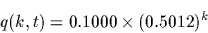Next: The BackDynProg Function Up: Dynamic Programming Previous: Dynamic Programming

## The DynProg Function

We want to find the alignment between the following two amino acid sequences that yields the highest score. For this example, we will use a Dayhoff matrix computed at PAM 250.

> CreateDayMatrix(logPAM1, 250);     # compute the Dayhoff matrix DM
> x := 'TCIYGH';
> y := 'TWMRH';

The function DynProg performs dynamic programming in Darwin.

Calling Sequences:
DynProg( seq1, seq2, DM)
DynProg( seq1, seq2, DM, l1, l2)
Parameters:
 seq1, seq2 : string DM : DayMatrix l1, l2 : posint

Returns: list - three elements (1) score, (2), length of match for sequence 1 and length of match for sequence 2.

Synposis: If no lengths are given as arguments ( l1, l2are not passed), the DynProg function computes the highest similarity score for the two sequences (or two subsequences of seq1 and seq2). This is referred to as the best global alignment.

If the lengths are given as arguments, then DynProg finds the alignment of length max(l1, l2) with maximum score. The alignment is guaranteed to use l1 elements of seq1 and l2 of seq2. This is referred to as the best local alignment.

We begin by tracing through an alignment of sequences x and y. First, we ask Darwin to compute the maximum local alignment (we omit the lengths of x and y.

> DynProg(x, y, DM);
[3.9785, 3, 3]

The score of the alignment is 18.0274 and it uses only 3 amino acid from both x and y. Although DynProg does not gives us the alignment, we can deduce easily what matches occured. The best way to visualize the alignment process is to build a matrix of dimension length(x)+1 by length(y)+1.

Imagine a beautiful, aesthetic sequence of arrays showing dynamic programming performing an elegant dance over the strings x and y. This will be filled in later.

The overall score for the alignment is calculated as follows:The DynProg routine judiciously decided to cut the alignment at the third position because the cost of matching amino acid Y against amino acid R is -1.8160 and matching H against G is -1.4169. Inclusion of either or both matches decrease the overall score.
    x:    T     C      I      Y      G    H
y:    T     W      M      R      H
2.52  -1.02  2.48  -1.82  -1.42


The remaining alternative is to insert a gap somewhere in the sequence. However, any such gap will incur a penalty of at least DM[FixedDel]=-19.8137. It is easy to verify that all of the following alignments score poorer than the alignment which keeps only the first three bases.

Alternative #1:
x:    T     C      I      Y      G      H
y:    T     W      M             R      H
2.52  -1.02  2.48         -1.00   6.05    -19.8 (gap)

Alternative #2:
x:    T     C      I      Y      G      H
y:    T     W      M                    R
2.52  -1.02  2.48                 0.62    -21.2100 (gap)

Alternative #3:
x:    T     C      I      Y      G      H
y:    T     W      M      R             H
2.52  -1.02  2.48  -1.82          6.05    -19.8 (gap)

Alternative #4:
x:    T     C      I             Y
y:    T     W      M      R      H
2.52  -1.02  2.48  -1.82   2.1697         -19.8 (gap)Next: The BackDynProg Function Up: Dynamic Programming Previous: Dynamic Programming
Gaston Gonnet
1998-09-15